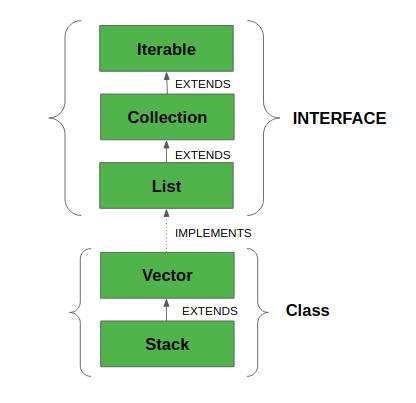# Stack Class in Java

Java Collection framework provides a Stack class which models and implements Stack data structure. The class is based on the basic principle of last-in-first-out. In addition to the basic push and pop operations, the class provides three more functions of empty, search and peek. The class can also be said to extend Vector and treats the class as a stack with the five mentioned functions. The class can also be referred to as the subclass of Vector.
This diagram shows the hierarchy of Stack class:The class supports one default constructor Stack() which is used to create an empty stack.
Below program shows few basic operations provided by the Stack class:

 `// Java code for stack implementation ` ` `  `import` `java.io.*; ` `import` `java.util.*; ` ` `  `class` `Test ` `{    ` `    ``// Pushing element on the top of the stack ` `    ``static` `void` `stack_push(Stack stack) ` `    ``{ ` `        ``for``(``int` `i = ``0``; i < ``5``; i++) ` `        ``{ ` `            ``stack.push(i); ` `        ``} ` `    ``} ` `     `  `    ``// Popping element from the top of the stack ` `    ``static` `void` `stack_pop(Stack stack) ` `    ``{ ` `        ``System.out.println(``"Pop :"``); ` ` `  `        ``for``(``int` `i = ``0``; i < ``5``; i++) ` `        ``{ ` `            ``Integer y = (Integer) stack.pop(); ` `            ``System.out.println(y); ` `        ``} ` `    ``} ` ` `  `    ``// Displaying element on the top of the stack ` `    ``static` `void` `stack_peek(Stack stack) ` `    ``{ ` `        ``Integer element = (Integer) stack.peek(); ` `        ``System.out.println(``"Element on stack top : "` `+ element); ` `    ``} ` `     `  `    ``// Searching element in the stack ` `    ``static` `void` `stack_search(Stack stack, ``int` `element) ` `    ``{ ` `        ``Integer pos = (Integer) stack.search(element); ` ` `  `        ``if``(pos == -``1``) ` `            ``System.out.println(``"Element not found"``); ` `        ``else` `            ``System.out.println(``"Element is found at position "` `+ pos); ` `    ``} ` ` `  ` `  `    ``public` `static` `void` `main (String[] args) ` `    ``{ ` `        ``Stack stack = ``new` `Stack(); ` ` `  `        ``stack_push(stack); ` `        ``stack_pop(stack); ` `        ``stack_push(stack); ` `        ``stack_peek(stack); ` `        ``stack_search(stack, ``2``); ` `        ``stack_search(stack, ``6``); ` `    ``} ` `} `

Output:

```Pop :
4
3
2
1
0
Element on stack top : 4
Element is found at position 3
```

Methods in Stack class

1. Object push(Object element) : Pushes an element on the top of the stack.
2. Object pop() : Removes and returns the top element of the stack. An ‘EmptyStackException’ exception is thrown if we call pop() when the invoking stack is empty.
3. Object peek() : Returns the element on the top of the stack, but does not remove it.
4. boolean empty() : It returns true if nothing is on the top of the stack. Else, returns false.
5. int search(Object element) : It determines whether an object exists in the stack. If the element is found, it returns the position of the element from the top of the stack. Else, it returns -1.My Personal Notes arrow_drop_up

Improved By : Chinmoy Lenka

Article Tags :

34

Please write to us at contribute@geeksforgeeks.org to report any issue with the above content.